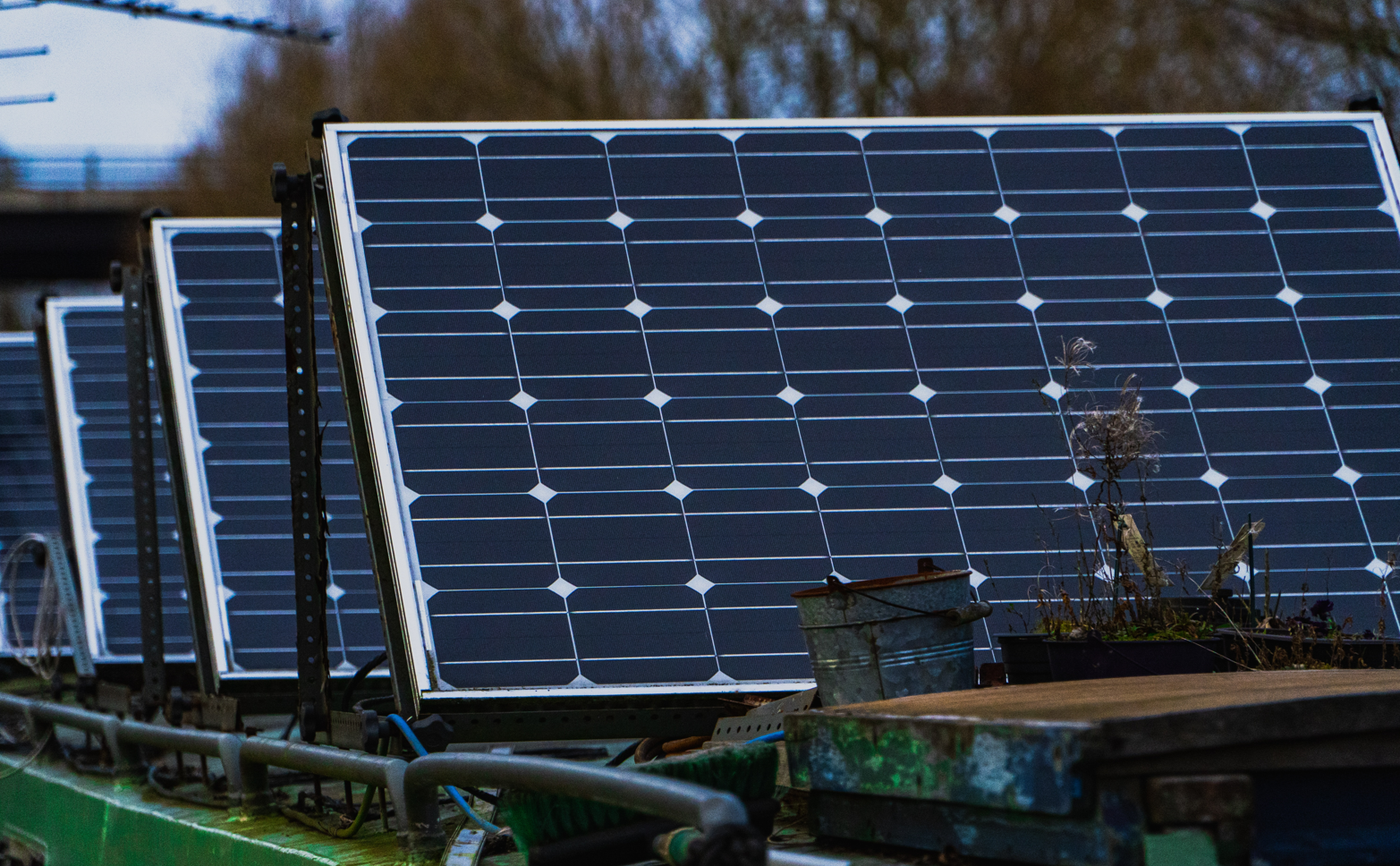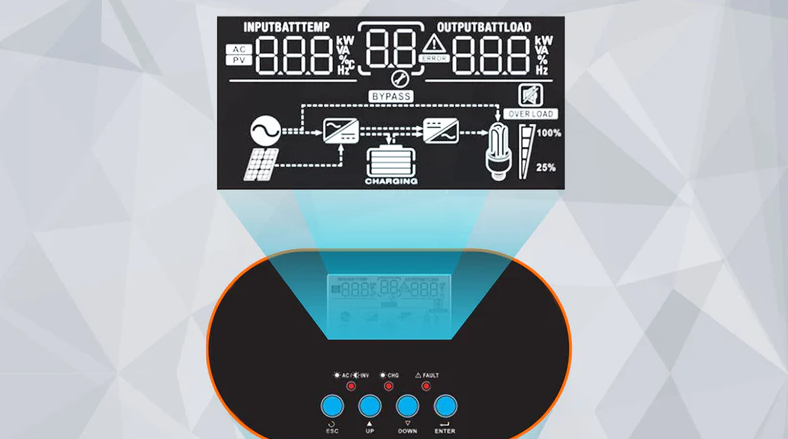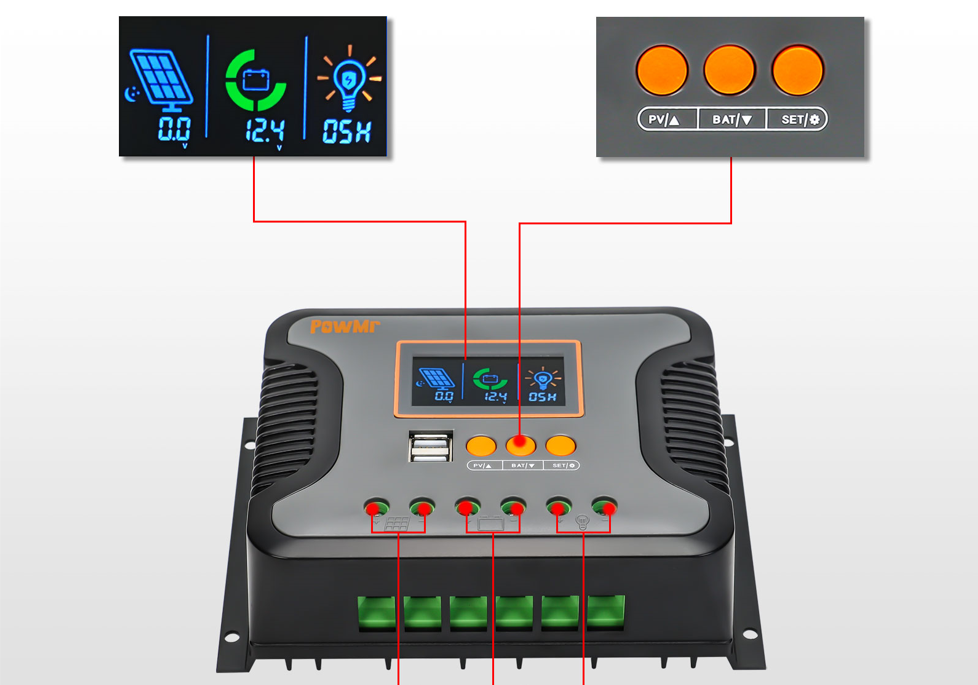# What can work with a 300w solar panel?A 300 watt solar panel with full irradiance will run on a constant 270 watt AC load, taking into account 10% inverter losses. This includes appliances like blenders, desktop computers, vacuum cleaners, and treadmills. A 300 watt solar panel will also run a small fridge with a 120 Ah lithium battery.

## How much power does a 300 watt solar panel produce?

Before calculating what will work with a 300-watt solar panel, we need to understand the power in watt-hours it can produce and under what conditions.

I will focus on the energy in watt-hours that a 300-watt solar panel can generate, rather than the instantaneous energy in watts. This is a much more useful value for matching panel output to load.

## 300 watt solar panel specifications

The important electrical specifications of the solar panel are:

• Open Circuit Voltage (Voc): Measured with a multimeter on the + and - leads
• Short-circuit current (Isc): measured with an online multimeter with short-circuited cables
• Peak Power Voltage (Vmp) – The volts at which peak power is generated
• Peak Power Current (Imp): The current that flows when peak power is generated

## How many kwh does a 300 watt solar panel produce?

With an average irradiance value of 4 peak hours of sunshine, a 300-watt solar panel produces 1.2 kilowatt-hours (kWh) of electrical energy per day, or 438kWh per year. The exact amount will vary depending on the location of the irradiance. If you supply AC appliances, deduct at least 10% of the inverter losses, depending on the size and efficiency of the inverter.

You will come across the term Maximum Power Point over and over again as you research solar power generation. This is when the panel volts and current are at optimum values for maximum power.

Power (watts) = volts x amps

This only happens under certain conditions:

• when irradiation is sufficient (how much solar energy is available)
• when load characteristics match panel requirements

### Solar irradiation and power output

The output of the solar panel is highly dependent on irradiance and varies greatly during the day: the maximum in a few hours around noon and very low in the early morning or evening.

Because of this, solar professionals talk in terms of average hours of peak irradiance in a day and use this to calculate the average power a panel delivers over time.

### How much power will a 300 watt solar panel produce?

In terms of energy, the amount of power can be calculated in this way:

Maximum Panel Rating x Peak Sun Hours/Day = Watt-hours per day

If we take Houston as an example, then a 300 watt solar panel would generate:

300 watts x 4,254 = 1,276kWh/day = 465.7kWh/year

## 300 watt solar panel how many amps?

The maximum amps of a 300 watt solar panel are called Imp (current at maximum power) and are provided by the manufacturer on the specification sheet. An average current is 9.5 amps DC for a 300 watt solar panel with a Voc of 42 volts. The equivalent current of an AC appliance (US) is approximately 3 amps.

The easiest way to know how many amps a 300-watt solar panel can deliver is to read the spec sheet. An average value is approximately 9.5 amps DC.

In terms of AC current, we must eliminate inverter losses of at least 10 percent and assume the panel is delivering full power.

If Vmp is 36 volts, then for a US AC load we need to reduce the DC current by the difference in DC/AC voltage. A simple equation could be:

AC Amps = DC Amps x 36/120 = 9.5 x 0.3 = 2.85 AC Amps

Remember that inverter efficiency varies with load and I'm guessing it's 90 percent when fully loaded. However, this also depends on the size of the inverter used.

Final Maximum AC Charge Current = 2.85 – (2.85*10/100) = 2.56 AC Amps

### What size inverter for a 300 watt solar panel?

At first glance, you'd think the answer is obviously 300 watts, but it's always better to overestimate your needs for a couple of reasons.

Let's say, for example, that you were using a fridge or freezer. It can operate quite comfortably in full sun, as the average refrigerator runs at 40 to 100 watts continuously.

However, when the compressor runs and the motor starts, it consumes up to 3 times more power due to overcurrent.

The 300 watt solar panel output may not be enough to supply this load, especially if the panel is not running at full power, which is usually the case, except for 4-5 hours around noon.

In that case a battery is essential and would be coupled to the 300 watt solar panels using a solar charge controller.

## What size charge controller for a 300 watt panel?

The average current produced by a 300 watt solar panel is between 9 and 9.5 amps, so a 10 amp solar charge controller works well.

However, it would be wise to upgrade to a 30 or even 60A model in case you want to increase solar capacity later on.

Most MPPT controllers have an input voltage rating of 60 volts, so a 300 volt solar panel with Voc between 40 and 44 volts will work.

## Can a 300 watt solar panel charge a 12 volt battery?

A 300 watt solar panel can charge a 12-volt battery and the time it takes depends on the state of discharge of the battery and the level of irradiation at the location of the solar panel.

With 5 peak hours of sun irradiation per day, a 300-watt solar panel will produce 1,500 watt-hours per day.

A 100 Ah 12 volt battery is equivalent to 1200 watt-hours, so a 300 watt solar panel with an MPPT solar controller will recharge a fully discharged 100 Ah 12 volt battery in less than 5 hours.

However, it is very rare for a battery to be completely discharged. A deep-cycle lead-acid battery typically discharges to 50 percent of its full capacity, so a 300-watt solar panel kit would recharge it in less than 2.5 hours.